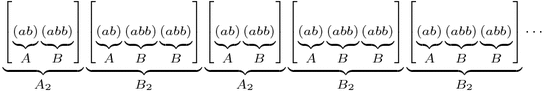# The “Fibonacci”est String

The celebrated Fibonacci Sequence is defined by setting each term equal to the sum of the two previous terms, starting at $$F_0=0$$ and $$F_1=1$$. So it continues like this:

0, 1, 1, 2, 3, 5, 8, 13, 21, 34, 55, 89, 144, 233, 377, …

What’s the relation to last week‘s infinite jump sequence,

a b a b b a b a b b a b b a b a b b a b a b b a b b a b a b b a b b … ?

Let’s find out!

Recall from last week that we can group our sequence into blocks of $$A=(ab)$$ and $$B=(abb)$$, and that the pattern of “short” and “long” blocks is the same as the pattern of as and bs in the original sequence:This means we can do this grouping again! Specifically, we can group our sequence into “blocks of blocks”, or level 2 blocks, that look like $$A_2=[(ab)(abb)]$$ and $$B_2=[(ab)(abb)(abb)]$$:Furthermore, the pattern of short and long level 2 blocks is still the same as the original jump sequence! So we can keep going, grouping our sequence into level 3 blocks $$A_3=A_2 B_2$$ and $$B_3=A_2 B_2 B_2$$, level 4 blocks $$A_4=A_3 B_3$$ and $$B_4=A_3 B_3 B_3$$, and so on, thus finding longer and longer patterns that fill up our sequence. Here’s level 3:One natural question is: what are the lengths of these blocks? Let’s make a table:

n An Bn An Length Bn Length
0 a b 1 1
1 ab abb 2 3
2 ababb ababbabb 5 8
3 ababbababbabb ababbababbabbababbabb 13 21

The lengths are exactly the Fibonacci numbers! (Prove this!) Furthermore, how many as and bs does each block have? Here’s another table:

n An # of as # of bs Bn # of as # of bs
0 a 1 0 b 0 1
1 ab 1 1 abb 1 2
2 ababb 2 3 ababbabb 3 5
3 ababbababbabb 5 8 ababbababbabbababbabb 8 13

Again the Fibonacci numbers! This gives us another way to compute the ratio of as to bs in the jump sequence: The ratios of bs to as in blocks An and Bn are $$F_{2n}/F_{2n-1}$$ and $$F_{2n+1}/F_{2n}$$ respectively, so as n gets larger these ratios should both approach last week’s answer, the golden ratio $$\phi$$.

OK, so our jump sequence is very “Fibonacci”y: it has blocks of Fibonacci lengths with Fibonacci proportions of as and bs. How can it get any “Fibonacci”er than that? Here’s how: Define a different infinite string using the same definition as the Fibonacci numbers themselves: write $$W_0=0$$, $$W_1=1$$, and after that, each string is formed by writing the previous two next to each other: $$W_n=W_{n-1}W_{n-2}$$. The Ws continue like this:

0, 1, 10, 101, 10110, 10110101, 1011010110110, 101101011011010110101, …,

and in the limit they define some infinite string of 0s and 1s that, without a doubt, is as “Fibonacci”y as it gets. But wait; except for the first digit, this is exactly the same as our jump sequence with 0=a and 1=b!
- 1 0 1 1 0 1 0 1 1 0 1 1 0 1 0 1 1 0 1 0 1 1 0 1 1 0 1 0 1 1 0 1 1 ... a b a b b a b a b b a b b a b a b b a b a b b a b b a b a b b a b b ... This truly is the Fibonacci numbers reincarnated in a binary string.